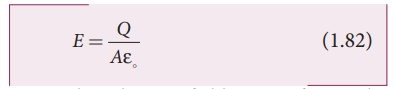Home | | Physics 12th Std | Capacitors

# Capacitors

Capacitor is a device used to store electric charge and electrical energy.

Capacitors

Capacitor is a device used to store electric charge and electrical energy. It consists of two conducting objects (usually plates or sheets) separated by some distance. Capacitors are widely used in many electronic circuits and have applications in many areas of science and technology.

A simple capacitor consists of two parallel metal plates separated by a small distance as shown in Figure 1.54 (a).When a capacitor is connected to a battery of potential difference V, the electrons are transferred from one plate to the other plate by battery so that one plate becomes negatively charged with a charge of –Q and the other plate positively charged with +Q. The potential difference between the plates is equivalent to the battery’s terminal voltage. This is shown in Figure 1.54(b). If the battery voltage is increased, the amount of charges stored in the plates also increase. In general, the charge stored in the capacitor is proportional to the potential difference between the plates.

Q V

so that Q = CV

where the C is the proportionality constant called capacitance. The capacitance C of a capacitor is defined as the ratio of the magnitude of charge on either of the conductor plates to the potential difference existing between the conductors.The SI unit of capacitance is coulomb per volt or farad (F) in honor of Michael Faraday. Farad is a very large unit of capacitance. In practice, capacitors are available in the range of microfarad (1µF = 10-6 F) to picofarad (1pf = 10-12 F). A capacitor is represented by the symbolNote that the total charge stored in the capacitor is zero (Q - Q = 0). When we say the capacitor stores charges, it means the amount of charge that can be stored in any one of the plates.

Nowadays there are capacitors available in various shapes (cylindrical, disk) and types (tantalum, ceramic and electrolytic), as shown in Figure 1.55. These capacitors are extensively used in various kinds of electronic circuits.## Capacitance of a parallel plate capacitorConsider a capacitor with two parallel plates each of cross-sectional area A and separated by a distance d as shown in Figure 1.56.The electric field between two infinite parallel plates is uniform and is given by E = σ / ε0 where σ is the surface charge density on the plates  (σ=Q/A) If the separation distance d is very much smaller than the size of the plate (d2 << A), then the above result is used even for finite–sized parallel plate capacitor.

The electric field between the plates isSince the electric field is uniform, the electric potential between the plates having separation d is given byTherefore the capacitance of the capacitor is given byFrom equation (1.84), it is evident that capacitance is directly proportional to the area of cross section and is inversely proportional to the distance between the plates. This can be understood from the following.

(i) If the area of cross-section of the capacitor plates is increased, more charges can be distributed for the same potential difference. As a result, the capacitance is increased.

(ii) If the distance d between the two plates is reduced, the potential difference between the plates (V = Ed) decreases with E constant. As a result, voltage difference between the terminals of the battery increases which in turn leads to an additional flow of charge to the plates from the battery, till the voltage on the capacitor equals to the battery’s terminal voltage. Suppose the distance is increased, the capacitor voltage increases and becomes greater than the battery voltage. Then, the charges flow from capacitor plates to battery till both voltages becomes equal.

Example 1.20

A parallel plate capacitor has square plates of side 5 cm and separated by a distance of 1 mm. (a) Calculate the capacitance of this capacitor. (b) If a 10 V battery is connected to the capacitor, what is the charge stored in any one of the plates? (The value of εo = 8.85 x 10-12 Nm2 C-2)

Solution

(a) The capacitance of the capacitor is= 221.2 ×10−13 F

C = 22 . 12 ×10−12 F = 22 .12 pF

(b) The charge stored in any one of the plates is Q = CV, Then

= 22 . 12 ×10−12 ×10 = 221.2 ×10−12 C = 221.2 pC

Study Material, Lecturing Notes, Assignment, Reference, Wiki description explanation, brief detail
12th Physics : Electrostatics : Capacitors |